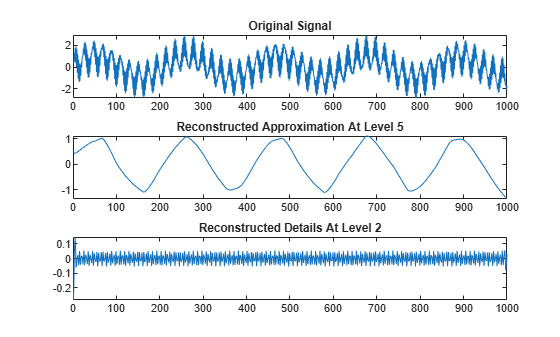wrcoef

Reconstruct single branch from 1-D wavelet coefficients

Description

x = wrcoef(type,c,l,wname) reconstructs the coefficients vector of type type based on the wavelet decomposition structure [c,l] of a 1-D signal (see wavedec for more information) using the wavelet specified by wname. The coefficients at the maximum decomposition level are reconstructed. The length of x is equal to the length of the original 1-D signal.

x = wrcoef(type,c,l,LoR,HiR) uses the reconstruction filters LoR and HiR.

example

x = wrcoef(___,n) reconstructs the coefficients at level n using any of the previous syntaxes.

Examples

collapse all

s = sumsin;

Perform a level 5 wavelet decomposition of the signal using the sym4 wavelet.

[c,l] = wavedec(s,5,'sym4');

Reconstruct the approximation coefficients at level 5 from the wavelet decomposition structure [c,l].

a5 = wrcoef('a',c,l,'sym4');

Reconstruct the detail coefficients at level 2.

d2 = wrcoef('d',c,l,'sym4',2);

Plot the original signal and reconstructed coefficients.

subplot(3,1,1)
plot(s)
title('Original Signal')
subplot(3,1,2)
plot(a5)
title('Reconstructed Approximation At Level 5')
subplot(3,1,3)
plot(d2)
title('Reconstructed Details At Level 2')Input Arguments

collapse all

Coefficients to reconstruct, specified as 'a' or 'd', for approximation or detail coefficients, respectively.

Wavelet decomposition of a 1-D signal, specified as a real-valued vector. The vector contains the wavelet coefficients. The bookkeeping vector l contains the coefficients by level. See wavedec.

Data Types: double | single

Bookkeeping vector, specified as a vector of positive integers. The bookkeeping vector is used to parse the coefficients in the wavelet decomposition c by level. See wavedec.

Data Types: double | single

Analyzing wavelet used to create the wavelet decomposition structure [c,l], specified as a character vector or string scalar. wrcoef supports only orthogonal or biorthogonal wavelets. See wfilters.

Wavelet reconstruction filters, specified as a pair of even-length real-valued vectors. LoR is the lowpass reconstruction filter, and HiR is the highpass reconstruction filter. The lengths of LoR and HiR must be equal. See wfilters for additional information.

Coefficients level, specified as a nonnegative integer. When type is 'a', n is allowed to be 0. Otherwise, n is a strictly positive integer such that n ≤ length(l)-2. The default value of n is length(l)-2.